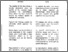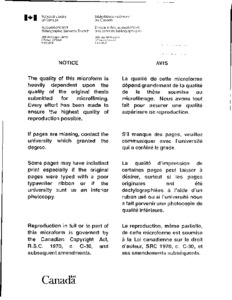Title:

# Chargino-neutralino production in pp̄-collisions for the left-right supersymmetric model

Saif, Harith N (1992) Chargino-neutralino production in pp̄-collisions for the left-right supersymmetric model. PhD thesis, Concordia University.Preview Text (application/pdf) NN84666.pdf 4MB

## Abstract

An extension of the supersymmetric standard model to the supersymmetric SU(2)$\sb{\rm L}$ x SU(2)$\sb{\rm R}$ x U(1)$\sb{\rm B-L}$ model is considered. The gauge group contains bi-doublet and triplet Higgs fields. In this work we have considered chargino (charged gauginos mixed with higgsinos) and neutralino (neutral gauginos mixed with higgsinos) eigenstates for the left-right supersymmetric (L-R SUSY) model. After mixing, in the minimal supersymmetric model (MSSM), there are two charginos and four neutralinos. In the L-R supersymmetric model, in addition to four charginos there are three neutralinos generated from the first symmetry breaking, and four neutralinos generates from the second symmetry breaking. The mixings are in general model dependent. In the minimal SUSY and L-R SUSY models, these mixings can be parameterized in terms of a few parameters. We find analytical expressions and numerical solutions for the mass eigenstates with some restrictions on the L-R parameters. We also investigate the possibility of detecting chargino and neutralino production in pp-collisions at CDF, namely $\rm p\bar{p}\to W\sbsp{L,R}{-} + X\to\ \sbsp{\chi\sb{j}}{\sim\sb-}\sbsp{\chi\sb{i}}{\sim0} + X.$ The expressions for these inclusive cross-sections are solved numerically, using parton model distributions. We have used $\rm \tilde{M}\sb\chi -= 45\ GeV,$ tan$\beta$ = 1 and a lower bound on the lightest supersymmetric particle (LSP) mass, $\tilde\chi$ = 14 GeV. Finally we take $\rm M\sb{WR}\ge 300$ GeV for $\rm g\simeq g\sb{L}\simeq g\sb{R}.$ In the pp-center of mass frame we find the cross sections are: $\rm \sigma\sb{L}(p\bar{p}\to W\sbsp{L}{-} + X\to\sbsp{\chi\sb{j}}{\sim\sb-}\sbsp{\chi\sb{i}}{\sim0} + X)\approx0.11$ nb, and $\rm \sigma\sb{R}(p\bar{p}\to W\sbsp{R}{-} + X\to\sbsp{\chi\sb{j}}{\sim\sb-}\sbsp{\chi\sb{i}}{\sim0} + X)\approx4.6$ pb, and thus $\rm \sigma\sb{L}\ge24\sigma\sb{R}$ at $\sqrt{\rm s}$ = 1.8 TeV. Cross sections are also given for larger values of the center of mass energy $\sqrt{\rm s}$ up to those available at the SSC. The results are compared with the prompt-lepton background of the $\rm W\sbsp{L,R}{-}$ decays from $\rm p\bar{p}\to W\sbsp{L,R}{-} + X\to\ell\sp-\nu\sb{\ell L,R} + X.$ Both decays for $\rm W\sbsp{L,R}{-}$-bosons show Jacobian peaks for $\rm p\sb{T} = \sqrt{\{s}}/2\simeq M\sb{WL}/2\simeq40$ GeV $\rm (p\sb{T}\simeq 150$ GeV for M$\sb{\rm WR})$ at $\theta=90\sp\circ.$ Furthermore the chargino signature unlike the prompt-lepton background is symmetric under the Jacobian peak. We also exhibit the dependence of the angular distribution of the chargino on the c.m angle $\theta$ for $\rm p\sb{T} = 40$ GeV, 150 GeV at $\sqrt{\rm s} = 1.8$ TeV.

Divisions: Concordia University > Faculty of Arts and Science > Physics Thesis (PhD) Saif, Harith N xiv, 179 leaves : ill. ; 29 cm. Concordia University Ph. D. Physics 1992 Kalman, C QC 174.17 S9S24 1992 6093 Concordia University Library 03 Sep 2009 13:48 13 Jul 2020 20:01 https://concordiauniversity.on.worldcat....
All items in Spectrum are protected by copyright, with all rights reserved. The use of items is governed by Spectrum's terms of access.

Repository Staff Only: item control pageResearch related to the current document (at the CORE website)
Back to top